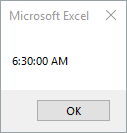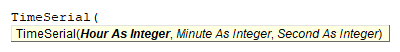# VBA TimeSerial Function

## TimeSerial Description

Returns a time given an hour, minute, and second value.

## Simple TimeSerial Examples

Here is a simple TimeSerial example:

``````Sub TimeSerial_Example()
MsgBox TimeSerial(6, 30, 0)
End Sub``````

This code will return 6:30:00 AM.## TimeSerial Syntax

In the VBA Editor, you can type  “TimeSerial(” to see the syntax for the TimeSerial Function:The TimeSerial function contains 3 arguments:

Hour: Numeric value between 0 (12:00 A.M.) and 23 (11:00 P.M.).

Minute: Numeric value representing a minute.

Second: Numeric value representing a second.

## Examples of Excel VBA TimeSerial Function

``MsgBox TimeSerial(16, 30, 0)``

Result: 4:30:00 PM

``MsgBox TimeSerial(16, -30, 10)``

Result: 3:30:10 PM

``MsgBox TimeSerial(16, -30, -10)``

Result: 3:29:50 PM

``MsgBox TimeSerial(-16, -30, -10)``

Result: 3:29:50 PM

Stop searching for VBA code online. Learn more about AutoMacro - A VBA Code Builder that allows beginners to code procedures from scratch with minimal coding knowledge and with many time-saving features for all users!